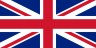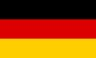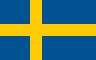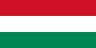GSAK (Geocaching Swiss Army Knife)Contents - Index

SqlGet (function)

SqlGet(sField,[nHandle]) : string

Optimized function to retrieve information from a SQLite query. Best used to iterate through a SQLite table.

This function should be used in conjunction with the SqlGet=Handle option of Sqlite(). It will allow you to retrieve the value of any field in the current SQLite query. Note: The return value of this function is *always* a string, and the full fidelity of the original string contents is preserved. You will need to convert date, numeric, and boolean values to their native format if you want to use functions that require the data in these formats. However, this is no different to the existing behaviour of the "Sql" action without SqlGet=Handle, as it too only ever returns string values.

sField - This is the name of the sqlite field in the database you want to get the value for.
nHandle - Optional, defaults to 0 if not used. Every SqlGet has an associated handle number (0..4) to allow for nesting of SqlGet loops. Each associated SqlGet command/function must use the same corresponding handle.

The following two code snippets are equivalent. However example 1 is much more efficient and will run about 6x faster.

Code 1

 \$status = sqlite("sql",\$_SqlGrid, "sqlget=0") while not(\$_sqleol)   \$data = sqlget("code") + sqlget("name") + sqlget("userdata")   sqlnext Endwhile

Code 2

 GoTo Position=Top While not(\$_Eol)   \$data = \$d_code + \$d_name + \$d_userdata   GoTo position=Next EndWhile GoTo position=Top

The following code will loop through 5 random caches in your database and show a message for each that lists the name and date of 5 associated logs
 \$Status = sqlite("sql","select * from caches limit 5","sqlget=0") While not(\$_SqlEol0)   \$code = SqlGet("Code")   \$data = \$Code + ": " + SqlGet("Name",0) + \$_CrLf   \$Status = sqlite("sql","select * from logs where lparent = '\$code' limit 5","sqlget=yes1")   While not(\$_SqlEol1)     \$data = \$data + "   " + SqlGet("lby",1) + ": " + SqlGet("ldate",1) + \$_CrLf     SqlNext Handle=1   Endwhile   SqlGetClose Handle=1   MsgOk Msg=\$data   SqlNext Handle=0 EndWhile SqlGetClose Handle=0

As the default handle is 0, the Handle for the outermost SqlGetClose,SqlNext,SqlGet and \$_SqlEol is not really required. So for brevity, the previous example can also be coded as:

 \$Status = sqlite("sql","select * from caches limit 5","sqlget=0") While not(\$_SqlEol)   \$code = SqlGet("Code")   \$data = \$Code + ": " + SqlGet("Name") + \$_CrLf   \$Status = sqlite("sql","select * from logs where lparent = '\$code' limit 5","sqlget=yes1")   While not(\$_SqlEol1)     \$data = \$data + "   " + SqlGet("lby",1) + ": " + SqlGet("ldate",1) + \$_CrLf     SqlNext Handle=1   Endwhile   SqlGetClose Handle=1   MsgOk Msg=\$data   SqlNext EndWhile SqlGetClose

Alpha List         Category List